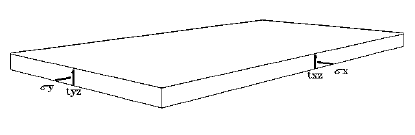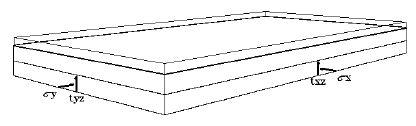The Rocscience International Conference 2021 Proceedings are now available. Read Now

# Geometry Overview

Rectangular Pillar

A Rectangular pillar is defined by it's length, width, and thickness (height), or x, y, and z values. These are the first 3 items entered in the Geometry section of the Pillar Information for Rigid, Elastic, or Voussoir analysis methods. (Depending on the relative magnitudes of x, y, and z, the term pillar, beam, or plate may be most applicable, as described at the end of this section. For convenience, the term pillar is used most often throughout the Online Help).

Plate with dimensions x = 10, y = 20, z = 1Plate with dimensions x = 10, y = 20, z = 1; overburden thickness = 1Plate with dimensions x = 10, y = 20, z = 1; overburden thickness = 1, water height = 2.5## Polygonal Pillar

A Polygonal pillar is defined by a at least three unique vertices (x, y), and thickness (i.e. height) (z) values. The polygon vertices can by input by selecting the Edit Coordinates button under the Geometry section in Pillar Information.

Non-vertical abutments can also be specified by changing the Abutment Trend and Abutment Plunge under the Geometry section in Pillar Information.

NOTE: Polygon Coordinates option and non-vertical abutments are only available in Rigid - Polygon analysis

## Pillars, Beams, and Plates

Depending on the relative magnitudes of x, y, and z, the term pillar, beam, or plate will most correctly describe the geometric model:

• A pillar refers to a model with a low span / depth ratio, the span being the shorter of the x and y dimensions.
• A beam refers to a model with one of the lateral dimensions substantially longer than the other, and a fairly high span / depth ratio (e.g. > 3). Note that the beam span is the short lateral dimension.
• A plate refers to a model where both lateral dimensions are of similar magnitude, and a fairly high span / depth ratio (e.g. > 3).

To model the roof of a long excavation (a beam), make one lateral dimension much longer than the other (e.g. x = 10, y = 100). A factor of 10 should suffice, although the user can specify any realistic dimensions (e.g. x = 10000, y = 10).

NOTE: The "long" and "short" lateral dimensions can be either the x or the y dimension. Make sure the corresponding lateral stresses are consistent with the chosen x and y directions.

## Is Pillar Permeable?

This option toggles between Yes and No.

No - Water height less than the pillar + overburden thickness will have no effect on the model whatsoever. If water height is greater than the pillar + overburden thickness, then the weight of the free water will have an effect as an extra deadload on the pillar.

Yes - Effect of water pressure on lateral effective stress will be taken into account. However, if water height is too high, negative effective stresses will results, and a warning message will appear when attempting to Compute.

## Face Dip

For a Voussoir analysis, the user can specify a dip angle (degrees from horizontal) for the excavation face (>=0 and < 90). Specifying an angle > 0 has the effect of lowering the effective gravitational driving force by a factor of cos(dip angle). Other than this, the analysis procedure is the same as for a zero dip angle.